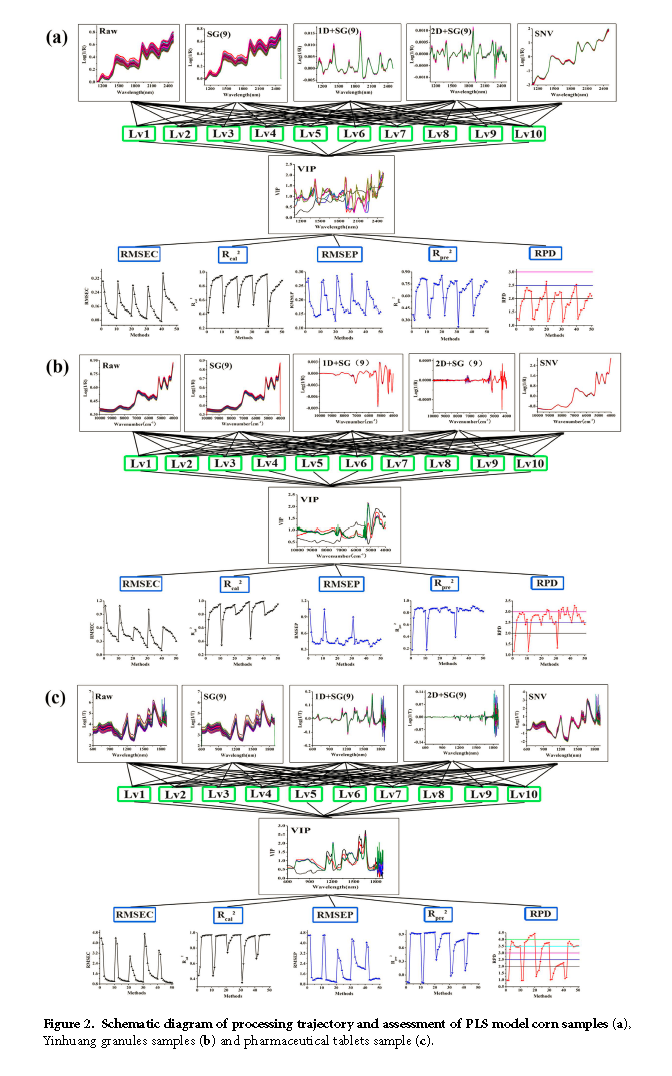## 赵娜同学在Scientific Reports发表了题为“Optimization of Parameter Selection for Partial Least Squares Model Development”的研究成果

Abstract: In multivariate calibration using a spectral dataset, it is difficult to optimize nonsystematic parameters in a quantitative model, i.e., spectral pretreatment, latent factors and variable selection. In this study, we describe a novel and systematic approach that uses a processing trajectory to select three parameters including different spectral pretreatments, variable importance in the projection (VIP) for variable selection and latent factors in the Partial Least-Square (PLS) model. The root mean square errors of calibration (RMSEC), the root mean square errors of prediction (RMSEP), the ratio of standard error of prediction to standard deviation (RPD), and the determination coefficient of calibration (Rcal 2) and validation (Rpre 2) were simultaneously assessed to optimize the best modeling path. We used three different near-infrared (NIR) datasets, which illustrated that there was more than one modeling path to ensure good modeling. The PLS model optimizes modeling parameters step-by-step, but the robust model described here demonstrates better efficiency than other published papers.